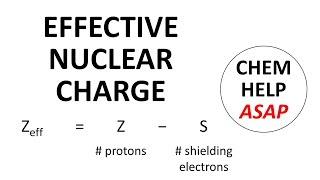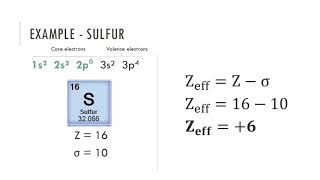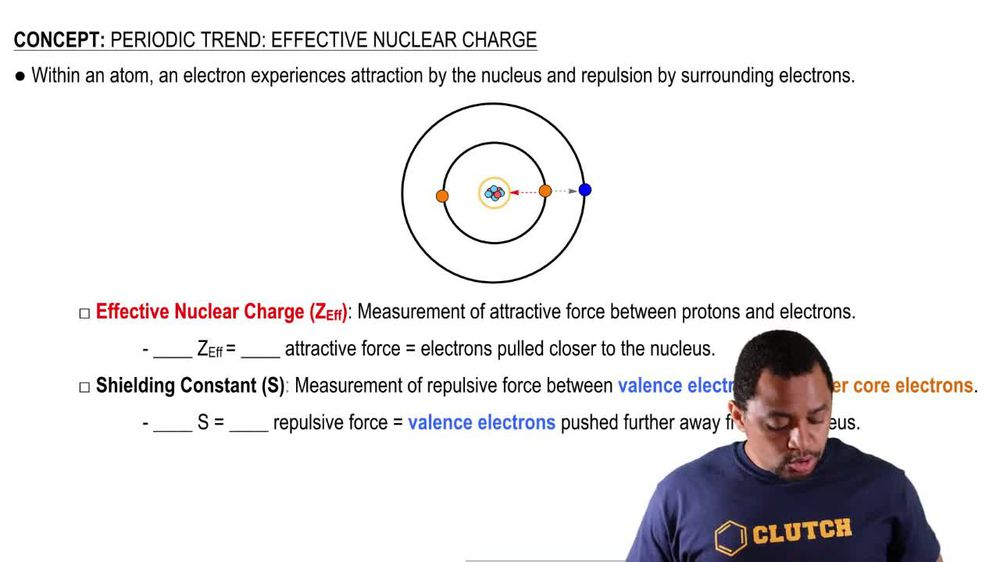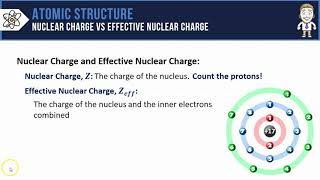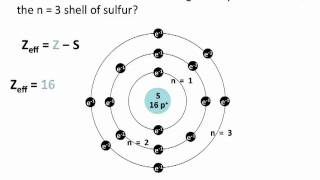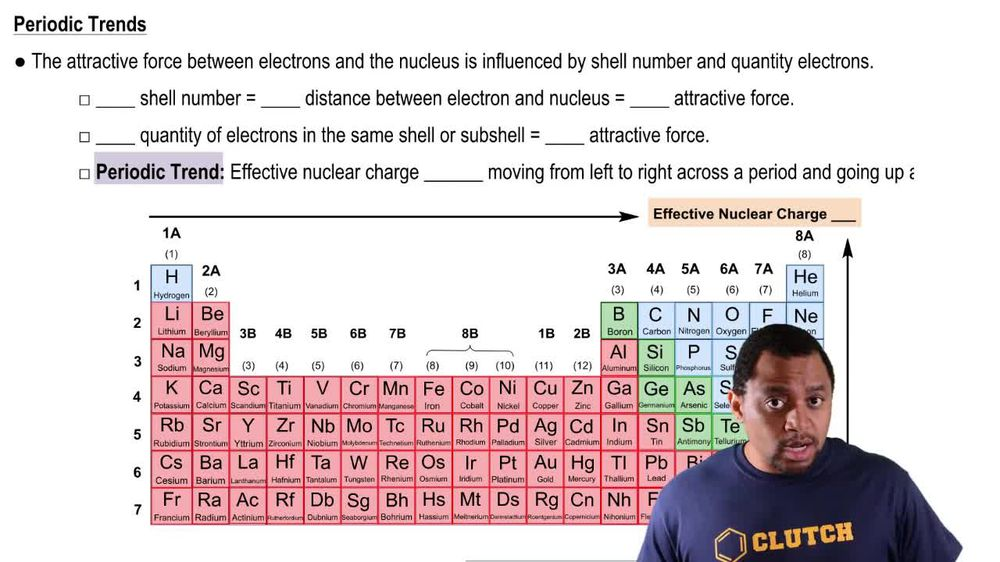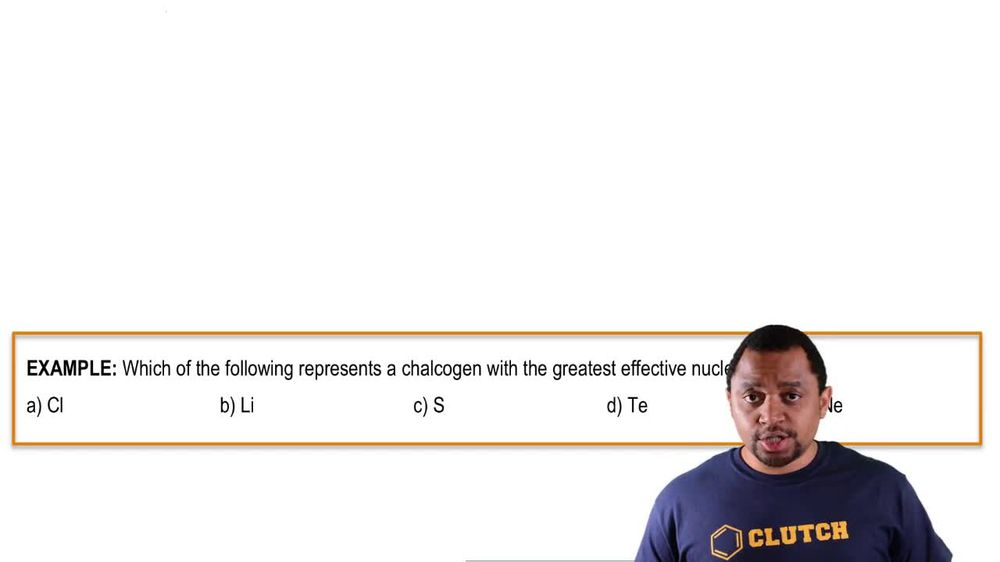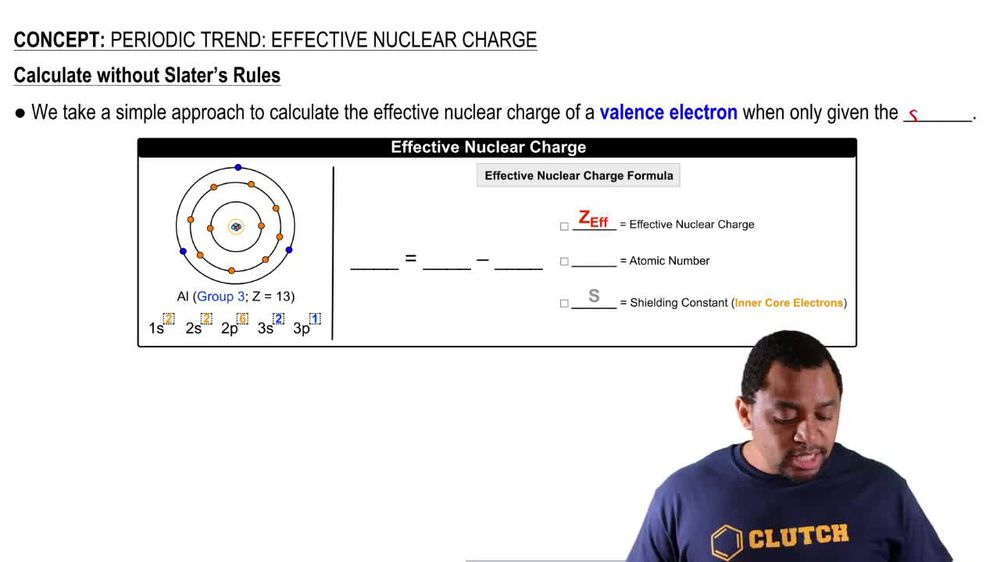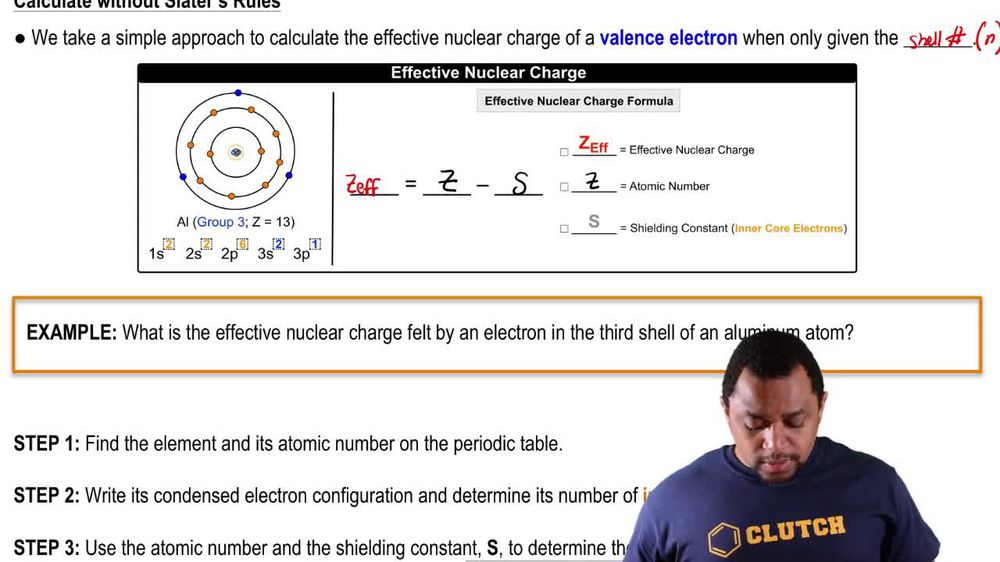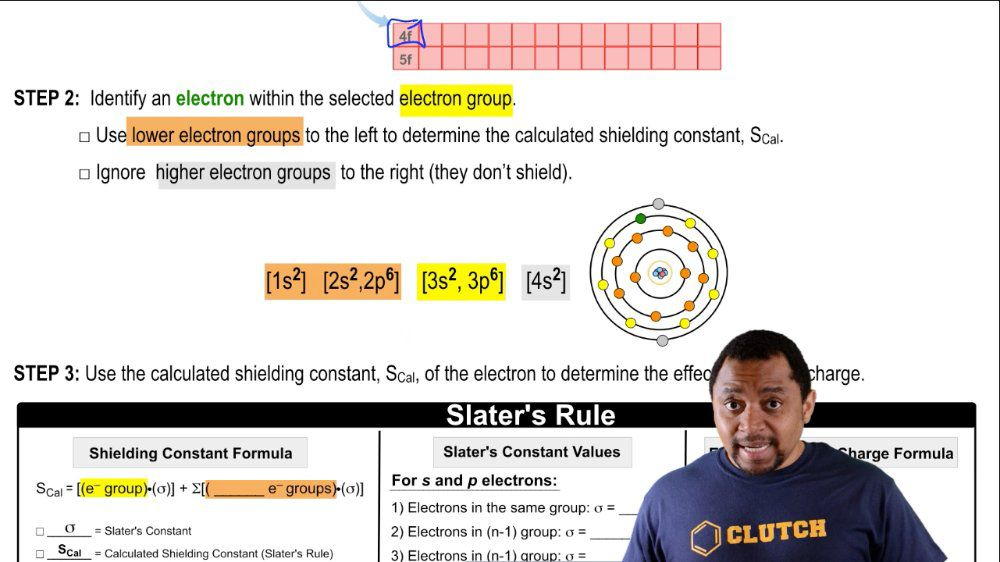Start typing, then use the up and down arrows to select an option from the list.
1. 10. Periodic Properties of the Elements2. Periodic Trend: Effective Nuclear Charge# Periodic Trend: Effective Nuclear Charge Example 3

by Jules Bruno
186 views
2
0
now here, we're going to use Slater's rule to determine our new, effective nuclear charge. Now, Slater's rule is just a system used to determine the effective nuclear charge off a specific electron within an orbital. So were given just more than the shell number for our electron. For this example, it says, using Slater's rules, calculate the effective nuclear charge of a three p orbital electron in calcium. So Step one is we're gonna group the electrons in an electron configuration in order of increasing and value and in this form, so calcium would be here on the periodic table if electron configuration will be one s two to s 22 p six three s 23 p six. We haven't gotten to three d with it. So we skipped three D for us to here. We have listed it in order in order of increasing and value. So this would be one because one here, this would be an equals two and equals three. Three d is also n equals three, but three, the S and P Orbital's air similar because they're in the same role of the periodic table. So we group them together three D is its own separate row. And if we have F, it would be its own separate thing as well. Then we have four s and four p, which are n equals four. Four d is here separate from for us and four p and then four f is also separate because it's in its own row. This would be five, and this would also be fine. Now this is important. We have to identify an electron within the selected electron group were asked to find the effect of nuclear charge of a three p electron. So it's here somewhere in here. We're gonna use the lower electron groups to the left to determine the calculated shielding constant because remember, the electrons in front are the ones protecting that electron from the full blast of the nucleus. Ignore higher electron groups. Those to the right, they don't shield us. So the electron we're looking at is this one. It's being shielded from the full effect of the nucleus by the electrons that are lower than it. These ones in orange. It's also being partially protected by the electrons. In the same, she'll visit the ones in yellow. Now we're gonna use the calculated show constant off the electron to determine the effect of nuclear charge, so the calculated shielding constant equals the electrons within your electron group. Times are are Slater's constant plus, adding up all of the lower electrons, the ones to the left of our electron times, their Slater's constant here. All of this will help us to calculate the shielding constant using Slater's role. All right, So how does this work well for S and P electrons? We're gonna say electrons in the same group, their slayers constant is equal to 35. If it's an end minus one group, then each electron is 85. And if its end minus two or greater than its 1. for DNF, if they're in the same group, it would be 35. And then if it's lower than that, then we would say here that it is 0.85. Then here it's s count equals your atomic number minus You're Slater's constant. So let's see how it work here and equals one here and equals to hear and equals three. And here and equals four. We're looking at one of these electrons here, so we'd say within there. There are a total off eight electrons, right? And we're looking at one of them. So that means there's seven other electrons in my same group with me. Each one of them is 10.35 which comes out to 2. and minus one. So one and value lower than me are these electrons here and there are eight of them total. Each of them contributes 0.85 when its end minus one. So that comes out to 6.80 Then finally, groups that our end minus two or greater, which is this one here. This one has two electrons within it. So that's two times 1.0 The total value here we get is 8.11 points are 11 point 25 So for calcium, we'd say that it's effective nuclear charge, which is s Cal equals it atomic number, which on the periodic table is 20 minus what we just found here for our sliders constant 11.25 Plugging all that in means that we're gonna feel in effect of 8.75 as the effective nuclear charge for calcium. So this is what the calcium electron within the three p electron orbital will feel in terms of the attractive pull of the nucleus, so it could be a little bit complicated. But this is the approach we have to take when they're giving us more specific information on an electron within a given orbital.